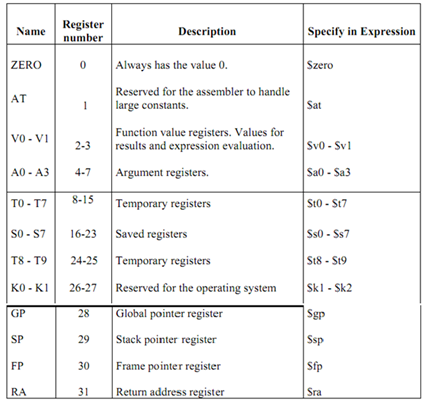## General registers in a processor, Computer Engineering

Assignment Help:

In this segment, we will give very brief details of registers of a RISC system known as MIPS.

MIPS is a register-to-register or load/store architecture and employs three address instructions for data manipulation. It is due to register-register operands that you can have more operands in an instruction of 32 bits because register address are smaller.

MIPS have 32 addressable registers = 25

Table given below shows MIPS general purpose registers.

MIPS register names start with a \$. There are two naming conventions:

MIPS register names start with a \$. There are two naming conventions:

• By number:

\$0   \$1   \$2    ...              \$31

• By (mostly) two-letter names like:

\$a0 - \$a3   \$t0 - \$t7   \$s0 - \$s7    \$gp    \$fp    \$sp    \$ra

Not all of these are general-purpose registers. The subsequent table explains how each general register is treated and actions you can take with each one of the registers.Thus all the computers have some registers. However how exactly is instruction execution related to registers?  To explore this idea let's first consider the idea of Micro-operations.

#### What is the significance of nonactionattribute, In general, all public proc...

In general, all public processes of a controller class are treated as action processes. If you require prevent this default behaviour, just decorate the public process with NonActi

#### Define the term- verification, Define the term- Verification It is a wa...

Define the term- Verification It is a way of preventing errors when data is copied from one medium to another (for instance from paper to disk/CD or from memory to DVD, etc.).

#### Major task of a computer to carry out instruction execution, The major task...

The major task of a computer is to carry out instruction execution. The key questions that can be asked in this respect are: (a) how are the instructions provided to computer? And

#### Why does ipv6 use separate extension headers, Why does IPV6 use separate ex...

Why does IPV6 use separate extension headers? Explain. The extension headers in Ipv6 are utilized for economy and extensibility. Partitioning the datagram functionality in sepa

#### Binary multiplication in matlab, source code for binary multiplication in m...

source code for binary multiplication in matlab

#### Explain about octal numbers system, Q. Explain about Octal Numbers system? ...

Q. Explain about Octal Numbers system? An octal system has 8 digits signified as 0,1,2,3,4,5,6,7. For finding equal decimal number of an octal number one has to find quantity o

#### Convert given grammar into GNF, S tends to aAS/a and A tends to SbA/SS/ba...

S tends to aAS/a and A tends to SbA/SS/ba

#### Solve out linear equations, Assume that you have been asked to solve proble...

Assume that you have been asked to solve problem with exact area constraints, the area error being no more than 1% for each department. What are the linear equations you would nee

#### What is the motivation for cell design, Question: a) Evary cell in a ce...

Question: a) Evary cell in a cellular network is assigned a band of frequencies. The allocated frequencies are divided into two types of channels. Indentify and describe each t

#### Explain a binary semaphore, Explain a binary semaphore with the help of an ...

Explain a binary semaphore with the help of an example? An abstract data type (ADT) is a semaphore which defines a nonnegative integer variable that apart from initialization i